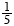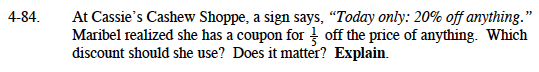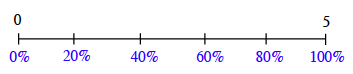### Home > MC1 > Chapter 4 > Lesson 4.3.1 > Problem4-84

4-84.

. At Cassie's Cashew Shoppe, a sign says, "Today only: 20% off anything." Maribel realized she has a coupon foroff the price of anything. Which discount should she use? Does it matter? Explain. Homework Help ✎$20\% \text{ is the same as }\frac{20}{100}.\text{ Can you use a Percent Ruler or number line to represent this?}$

$\text{Using the same ruler or number line to represent }\frac{1}{5}\text{ and 20\% might help you compare the portions.}$

$\text{Remember that one whole, or 100\%, is the same as }\frac{5}{5}.$

$\text{Your number line might resemble the one below. Can you place 20\% and }\frac{1}{5}?$When you plot the portions, what do you find? Remember to explain your answer!